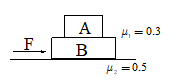Two block problem
Question

# In the figure, ${\mathrm{m}}_{\mathrm{A}}$ = 3kg and ${\mathrm{m}}_{\mathrm{B}}$ = 4 kg. For what minimum value of F, A starts slipping over B:(g = 10 $\mathrm{m}/{\mathrm{s}}^{2}$)Moderate
Solution

## maximum acceleration without slipping is 0.3 gTotal force required 0.3g(${\mathrm{m}}_{1}+{\mathrm{m}}_{2}$)+0.5(${\mathrm{m}}_{1}+{\mathrm{m}}_{2}$)g(${\mathrm{m}}_{1}+{\mathrm{m}}_{2}$)g (0.3+0.5)8(${\mathrm{m}}_{1}+{\mathrm{m}}_{2}$)=8(7)=56 N.

Get Instant Solutions